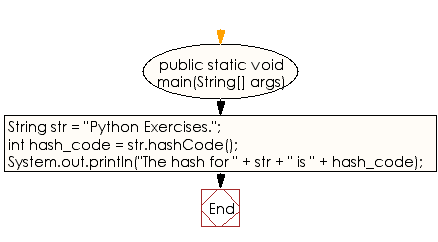﻿ Java exercises: Create a unique identifier of a given string - w3resource# Java String Exercises: Create a unique identifier of a given string

## Java String: Exercise-18 with Solution

Write a Java program to create a unique identifier of a given string.

Sample Solution:

Java Code:

``````public class Exercise18 {

public static void main(String[] args)
{
String str = "Python Exercises.";

// Get the hash code for the above string.
int hash_code = str.hashCode();

// Display the hash code.
System.out.println("The hash for " + str +
" is " + hash_code);
}
}
```
```

Sample Output:

```The hash for Python Exercises. is 863132599
```

Flowchart:Visualize Java code execution (Python Tutor):

Java Code Editor:

Improve this sample solution and post your code through Disqus

What is the difficulty level of this exercise?

﻿

## Java: Tips of the Day

Count the number of occurrences of a char in a String:

```int count = StringUtils.countMatches("a.b.c.d", ".");
```

Ref: https://bit.ly/2OTy6sG